# Determining CG Changes Caused by Modifying the Cargo (Part 3)

## Determining Changes in Weight and CG When the Aircraft Is Operated in Its Cargo Configuration

To determine changes in weight and CG when the aircraft is operated in its cargo configuration, the Beech 1900 is used as an example. Figure 1 illustrates the airplane configuration. Notice that the arm of each cargo section is the centroid of that section.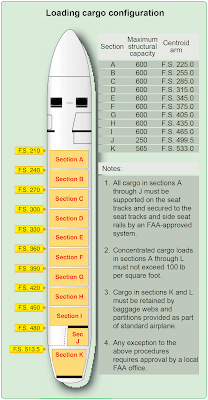Figure 1. Loading data for cargo configuration

The flight manifest of the Beech 1900 in the cargo configuration is illustrated in Figure 2. The BOW includes the pilots and their baggage and there is no separate item for them.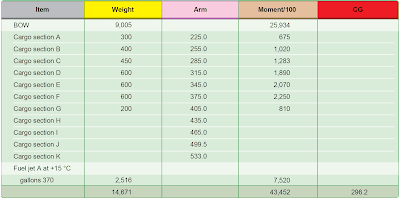Figure 2. Flight manifest of a Beech 1900 in the cargo configuration

At the standard temperature of 15 °C, the fuel weighs 6.8 pounds per gallon. Refer to Figure 3 to determine the weight and moment index of 370 gallons of Jet A fuel. The CG under these loading conditions is located at station 296.2.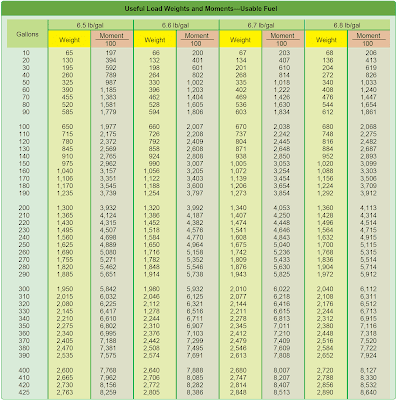Figure 3. Weights and moments—usable fuel

## Determining the CG Shift When Cargo Is Moved From One Section to Another

To calculate the CG when cargo is shifted from one section to another, use the formula found in Figure 4. If the cargo is moved forward, the CG is subtracted from the original CG. If the cargo is shifted aft, add the CG to the original.Figure 4. Shifting cargo from one section to another

## Determining the CG Shift When Cargo Is Added or Removed

To calculate the CG when cargo is added or removed, add or subtract the weight and moment index of the affected cargo to the original loading chart. Determine the new CG by dividing the new moment index by the new total weight, and multiply this by the reduction factor. [Figure 5]Figure 5. Determining the new CG by dividing the new moment index by the new total weight, multiplied by the reduction factor

## Determining Which Limits Are Exceeded

When preparing an aircraft for flight, consider all parameters and check to determine that no limits have been exceeded. Consider the parameters below, and determine which limit, if any, has been exceeded.
• The aircraft in this example has a basic empty weight of 9,005 pounds and a moment index of 25,934 pound inches/100.
• The crew weight is 340 pounds and its moment/100 is 439.
• The passengers and baggage have a weight of 3,950 pounds and a moment/100 of 13,221.
• The fuel is computed at 6.8 lb/gal. The ramp load is 340 gallons or 2,312 pounds. Fuel used for start and taxi is 20 gallons, or 136 pounds. Fuel remaining at landing is 100 gallons, or 680 pounds.
• Maximum takeoff weight is 16,600 pounds.
• Maximum zero fuel weight is 14,000 pounds.
• Maximum landing weight is 16,000 pounds.

Take these steps to determine which limit, if any, is exceeded:

1. Determine the zero fuel weight, which is the weight of the aircraft with all of the useful load except the fuel onboard. [Figure 6] The zero fuel weight of 13,295 pounds is less than the maximum of 14,000 pounds, so this parameter is acceptable.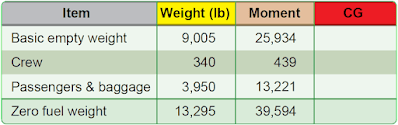Figure 6. Determining the zero fuel weight

2. Determine the takeoff weight and CG. The takeoff weight is the zero fuel weight plus the weight of the ramp load of fuel, minus the weight of the fuel used for start and taxi. The takeoff CG is the moment/100 divided by the weight, and then the result multiplied by 100. The takeoff weight of 15,471 pounds is below the maximum takeoff weight of 16,600 pounds, and a check of the weight and balance diagram shows that the CG at station 298.0 is also within limits. [Figure 7]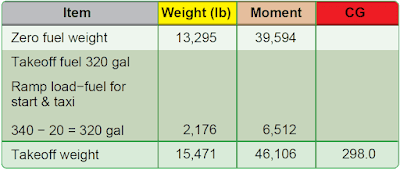Figure 7. Determining the takeoff weight and CG

3. Determine the landing weight and CG. This is the zero fuel weight plus the weight of fuel at landing. [Figure 8]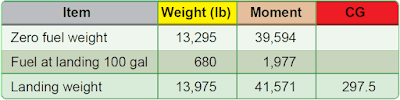Figure 8. Determining the landing weight and CG

The landing weight of 13,975 pounds is less than the maximum landing weight of 14,000 to 16,000 pounds. According to the weight and balance diagram, the landing CG at station 297.5 is also within limits.